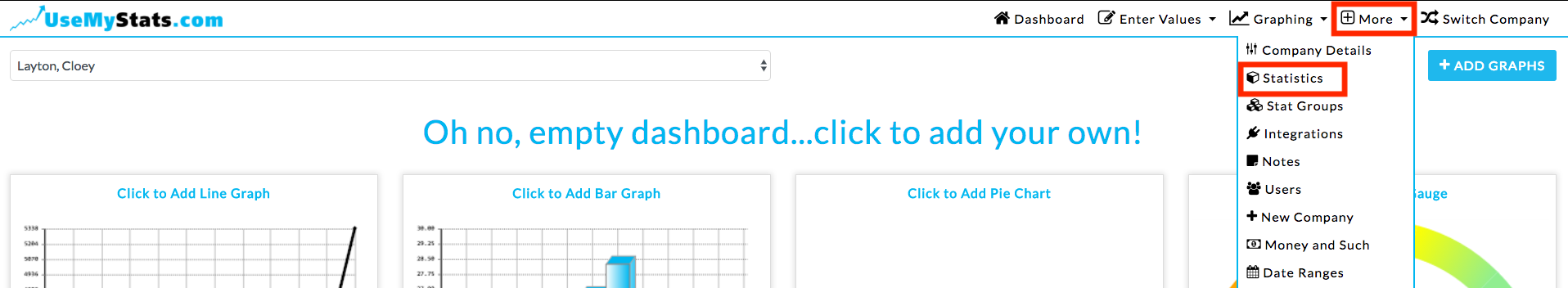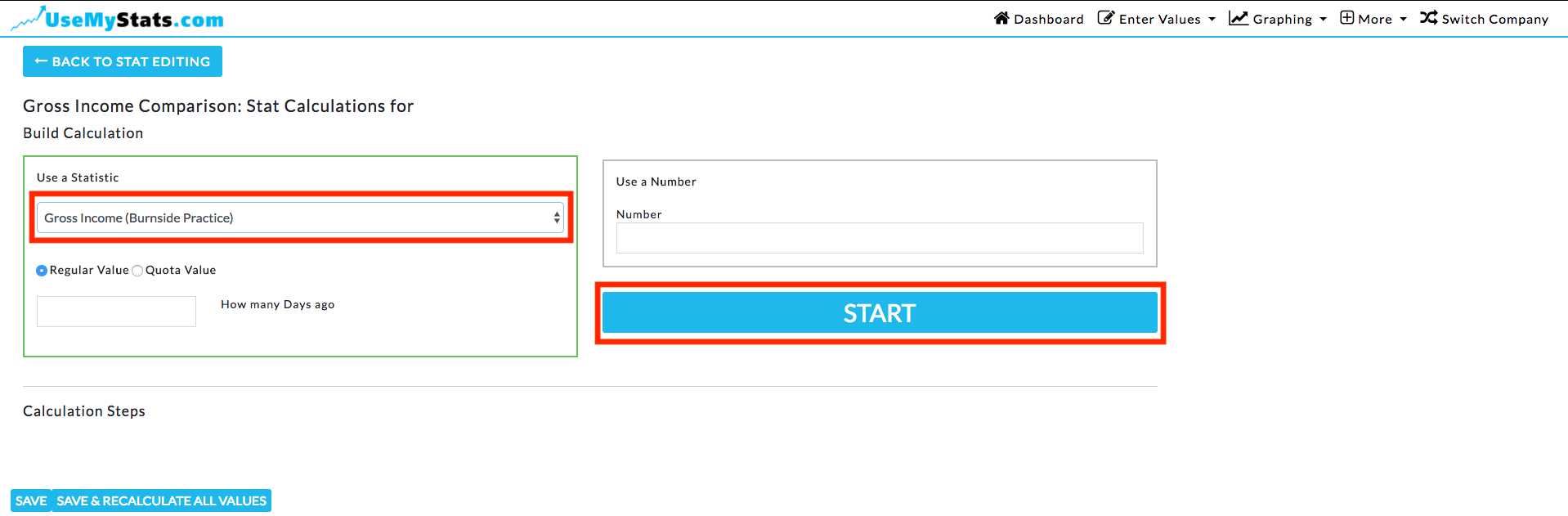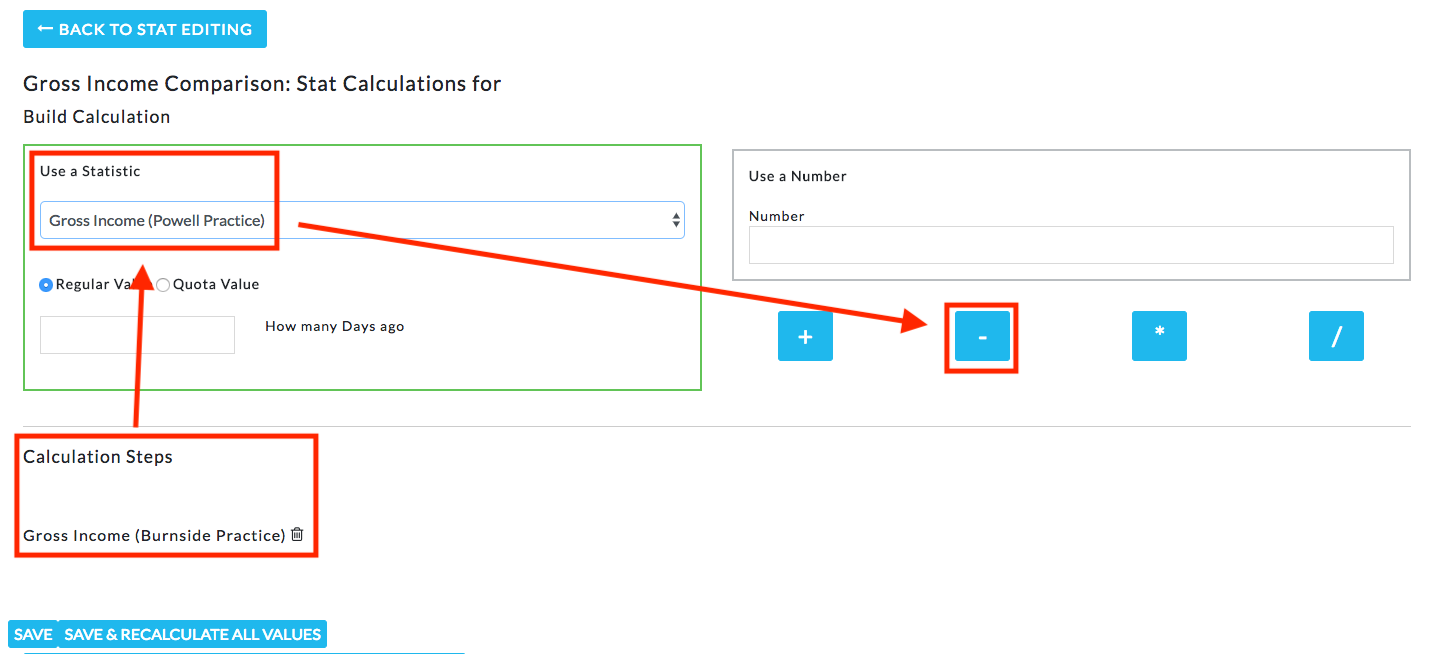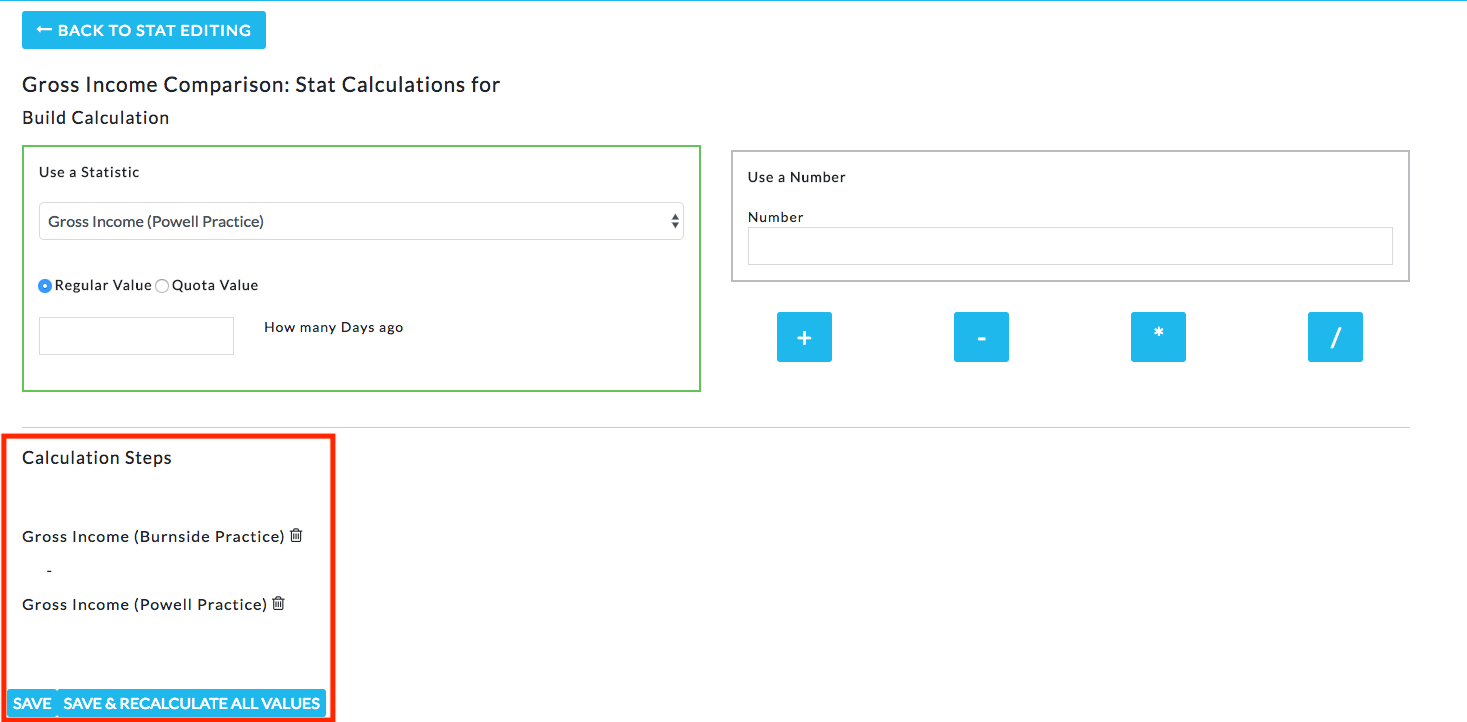Have you ever had a stat that you needed to calculate before you could enter the value? Perhaps you want to know the total hours worked this week, but that means you have to add fifty different employee's hours, by hand. Or you may need to find out the average gross income per employee. Instead of doing the math every time, take advantage of the automatic calculations feature!

There are three ways to calculate statistics on UseMyStats. This article cover calculations involving a direct relationship between two or more separate statistics, as in the examples above.

Once you are logged in, go to "More", then "Statistics".In this case, I'm going to set a calculation for Gross Income Comparison which will automatically subtract one practices gross income from the other. The first step is to create the statistic using the "Add Stat" button.Set the stat name and make sure the time period matches the time period on the statistics you want to use in the calculation, then change the Values option to "Calculation" and click "Set up Data".Now, I select the first statistic I need to use in the calculation - Gross Income, and click "Start".The first statistic I'm using now displays below under "Calculation Steps". I select the second statistic, Gross Income for my other Practice, and click the subtraction button.Both steps now show in the "Calculation Steps".Now if I use the regular save button, the calculation will kick in on the next time period where the Gross Income and Gross Expenses are entered - and Net Income will be automatically entered for you.

The Save & Recalculate All Values button will go back through any prior weeks where Gross Income and Gross Expenses were entered and enter Net Income for those weeks - then continue forward.